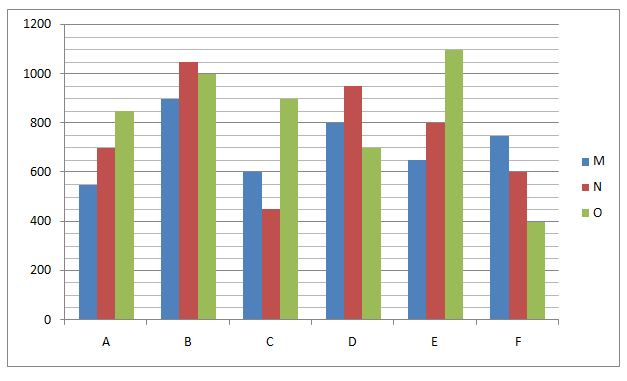# NIACL AO Mains– Quantitative Aptitude Questions Day- 14

Dear Readers, Bank Exam Race for the Year 2019 is already started, To enrich your preparation here we have providing new series of Practice Questions on Quantitative Aptitude – Section. Candidates those who are preparing for NIACL AO Mains 2019 Exams can practice these questions daily and make your preparation effective.

[WpProQuiz 5203]

Directions (Q. 1 – 5): What value should come in place of (?) in the following number series?

1) 13, 69, 31, 223, 57, ?

a) 478

b) 553

c) 521

d) 429

e) 392

2) 1056, 528, 352, 264, ?

a) 234

b) 256.8

c) 177

d) 189.5

e) 211.2

3) 2070, 345, 69, ? , 5.75, 2.875

a) 23.5

b) 32.25

c) 17.25

d) 28.5

e) 39.25

4) 115, 111, 231, 677, 2733, ?

a) 9563

b) 13629

c) 11757

d) 15215

e) 19421

5) 36, 188, 342, 499, 661, ?

a) 830

b) 956

c) 878

d) 924

e) 842

Directions (Q. 6 – 10): Study the following information carefully and answer the given questions:

The following bar graph shows the total number of different category flats (M, N and O) booked by various state people in the year 2018.6) Total number of M category flats booked by state A and C together is approximately what percentage of total number of O category flats booked by state B and E together?

a) 40 %

b) 70 %

c) 55 %

d) 85 %

e) 100 %

7) Out of the total number of O category flats booked by state B people, 28 % of the flats booked by financial employees. The remaining flats booked by Software employees and teachers in the ratio of 7: 5. Then find the ratio between the total number of financial employees booked the O category flats to that of total number of teachers booked the O category flats of state B? (Note: Only three category employees booked the flats)

a) 14: 15

b) 23: 26

c) 8: 11

d) 13: 17

e) None of these

8) Find the average number of O category flats booked by all the given state people together?

a) 750

b) 575

c) 700

d) 825

e) None of these

9) All the given category flats booked by state B people is approximately what percentage more/less than the all the given category flats booked by state F people?

a) 90 %

b) 70 %

c) 55 %

d) 105 %

e) 120 %

10) Find the difference between the total number of M and N category flats booked by state A and C people together to that of total number of M and O category flats booked by state D and F together?

a) 450

b) 600

c) 200

d) 350

e) None of these

Directions (1-5):

The pattern is, 32 + 4, 43 + 5, 52 + 6, 63 + 7, 72 + 8, 83 + 9

The pattern is, *(1/2), *(2/3), *(3/4), *(4/5),..

The pattern is, ÷ 6, ÷ 5, ÷ 4, ÷ 3, ÷ 2,…

The pattern is, *1 – 22, *2 + 32, *3 – 42, *4 + 52, *5 – 62,….

The difference of difference is, 2, 3, 5, 7, 11,… (Prime numbers)

Directions (6-10):

Total number of M category flats booked by state A and C together

= > 550 + 600 = 1150

Total number of O category flats booked by state B and E together

= > 1000 + 1100 = 2100

Required % = (1150/2100)*100 = 55 %

The total number of financial employees booked the O category flats of state B

= > 1000*(28/100) = 280

The total number of teachers booked the O category flats of state B

= > 720*(5/12) = 300

Required ratio = 280: 300 = 14: 15

The average number of O category flats booked by all the given state people together

= > (850 + 1000 + 900 + 700 + 1100 + 400)/6

= > 4950/6 = 825

All the given category flats booked by state B people

= > 900 + 1050 + 1000 = 2950

All the given category flats booked by state F people

= > 750 + 600 + 400 = 1750

Required % = [(2950 – 1750)/1750]*100 = 68.57 % = 70 %

The total number of M and N category flats booked by state A and C people together

= > 550 + 700 + 600 + 450 = 2300

The total number of M and O category flats booked by state D and F together

= > 800 + 700 + 750 + 400 = 2650

Required difference = 2650 – 2300 = 350